Study Materials

# NCERT Solutions for Class 10th Mathematics

Page 2 of 4

## Chapter 1. Real Numbers

### EXERCISE 1.2

Q1. Express each number as a product of its prime factors:
(i) 140

Solution: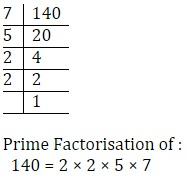= 22 × 5 × 7

(ii) 156

Solution: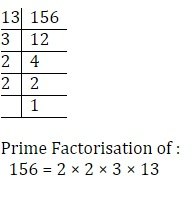= 22 × 3 × 13

(iii) 3825

Solution: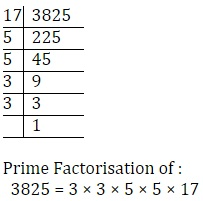= 32 × 52 × 17

(iv) 5005

Solution: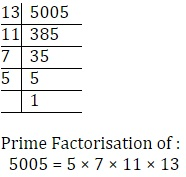= 5 × 7 × 11 × 13

(v) 7429

Solution: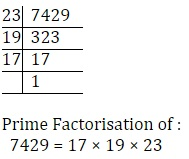Q2. Find the LCM and HCF of the following pairs of integers and verify that LCM × HCF = product of the two numbers.

(i) 26 and 91

Solution:

26 = 2 × 13

91 = 7 × 13

Common factors = 13

∴ HCF = 13

LCM = 2 × 7 × 13 = 182

Now varification,

product of the two numbers = LCM × HCF

N1 × N2LCM × HCF

26 × 91 = 13 × 182

2366 =  2366

Hence Varified,

(ii) 510 and 92

Solution:

510 = 2 × 3 × 5 × 17

92 = 2 × 2 × 23

Common factors = 2

∴ HCF = 2

LCM = 2 × 2 × 3 × 5 × 17 × 23 =  23460

Now varification,

product of the two numbers = LCM × HCF

N1 × N2 = LCM × HCF

510 × 92 = 2 × 23460

46920 =  46920

Hence Varified,

(iii) 336 and 54

Solution:

336 = 2 × 2 × 2 × 2 × 3 × 7

54 = 2 × 3 × 3 × 3

Common factors = 2 × 3

∴ HCF = 6

LCM = 2 × 2 × 2× 2 × 3 × 3 × 3 × 7 =  3024

Now varification,

product of the two numbers = LCM × HCF

N1 × N2 = LCM × HCF

336 × 54 = 6 × 3024

18144 =  18144

Hence Varified,

Q3. Find the LCM and HCF of the following integers by applying the prime factorisation method.

(i) 12, 15 and 21

Solution:

12 = 2 × 2 × 3

15 = 5 × 3

21 = 7 × 3

Common Factors = 3

HCF = 3

​LCM = 3 × 2 × 2 × 5 × 7 = 420

(ii) 17, 23 and 29

Solution:

17 = 1 × 17

23 = 1 × 23

29 = 1 × 29

HCF = 1

LCM = 17 × 23 × 29 = 11339

(iii) 8, 9 and 25

Solution:

8 = 2 × 2 × 2

9 = 3 × 3

25 = 5 × 5

There is no common factor except 1.

∴ HCF = 1

LCM = 2 × 2 × 2 × 3 × 3 × × 5

= 8 × 9 × 25

= 1800

Q4. Given that HCF (306, 657) = 9, find LCM (306, 657).

Solution:

HCF (306, 657) = 9

LCM × HCF = ​N1 × N2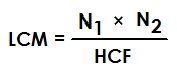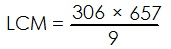LCM = 22338

Q5. Check whether 6n can end with the digit 0 for any natural number n.

Solution:

Prime factorisation of 6n = (2 × 3 )n

While, Any natural number which end with digit 0 has

the prime factorisation as form of (2 × 5 )n

Therefore, 6n will not end with digit 0.

Q6. Explain why 7 × 11 × 13 + 13 and 7 × 6 × 5 × 4 × 3 × 2 × 1 + 5 are composite numbers.

Solution:

Let A = 7 × 11 × 13 + 13

= 13 (7 × 11 + 1)

= 13 (77 + 1)

= 13 × 78

Hence this is composite number because It has at least one positive divisor other than one.

Similarily,

Let B = 7 × 6 × 5 × 4 × 3 × 2 × 1 + 5

= 5 (7 × 6 × 4 × 3 × 2 × 1 + 1)​

= 5 × (1008 + 1)

= 5  ×  1009

Hence this is also a composite number because It has at least one positive divisor other than one.

Q7. There is a circular path around a sports field. Sonia takes 18 minutes to drive one round of the field, while Ravi takes 12 minutes for the same. Suppose they both start at the same point and at the same time, and go in the same direction. After how many minutes will they meet again at the starting point?

Solution:

Sonia takes 18 minutes in one round.

Ravi takes 12 minutes in one round

they will meet again at the starting point after LCM(18, 12) minutes

18 = 2 × 3 × 3

12 = 2 × 2 × 3

HCF = 2 × 3 = 6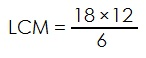= 36 minutes

Page 2 of 4

Chapter Contents: